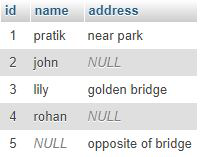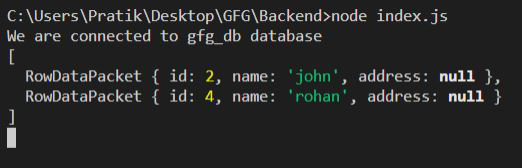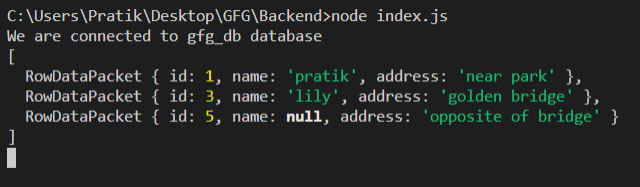GeeksforGeeks App
Open AppBrowser
Continue

# Node.js MySQL NULL Values

In this article, will learn to handle NULL values and make Query on the basis of NULL values.

Syntax:

`IS NULL;`
`IS NOT NULL;`

Return Value:

• ‘IS NULL’ returns the row in the column which contains one or more NULL values.
• ‘IS NOT NULL’ returns the row in the column which does not contains any NULL values.

Module Installation: Install the MySQL module using the following command.

`npm install mysql`

Database: Our SQL publishers table preview with sample data is shown below.Example 1:

## index.js

 `const mysql = require(``"mysql"``);`` ` `let db_con  = mysql.createConnection({``    ``host: ``"localhost"``,``    ``user: ``"root"``,``    ``password: ``''``,``    ``database: ``'gfg_db'``});`` ` `db_con.connect((err) => {``    ``if` `(err) {``      ``console.log(``"Database Connection Failed !!!"``, err);``      ``return``;``    ``}`` ` `    ``console.log(``"We are connected to gfg_db database"``);`` ` `    ``// Here is the query``    ``let query = ``"SELECT * FROM users WHERE address IS NULL"``;`` ` `    ``db_con.query(query, (err, rows) => {``        ``if``(err) ``throw` `err;`` ` `        ``console.log(rows);``    ``});``});`

Output:Example 2:

## index.js

 `const mysql = require(``"mysql"``);`` ` `let db_con  = mysql.createConnection({``    ``host: ``"localhost"``,``    ``user: ``"root"``,``    ``password: ``''``,``    ``database: ``'gfg_db'``});`` ` `db_con.connect((err) => {``    ``if` `(err) {``      ``console.log(``"Database Connection Failed !!!"``, err);``      ``return``;``    ``}`` ` `    ``console.log(``"We are connected to gfg_db database"``);`` ` `    ``// Here is the query``    ``let query = ``"SELECT * FROM users WHERE address IS NOT NULL"``;`` ` `    ``db_con.query(query, (err, rows) => {``        ``if``(err) ``throw` `err;`` ` `        ``console.log(rows);``    ``});``});`

Output:My Personal Notes arrow_drop_up
Related Tutorials